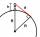# Sphere - problems

1. Cube in a sphereThe cube is inscribed in a sphere with volume 6116 cm3. Determine the length of the edges of a cube.
2. CubesOne cube is inscribed sphere and the other one described. Calculate difference of volumes of cubes, if the difference of surfaces in 257 mm2.
3. SphereSurface of the sphere is 2820 cm2, weight is 71 kg. What is its density?
4. MO SK/CZ Z9–I–3John had the ball that rolled into the pool and it swam in the water. Its highest point was 2 cm above the surface. Diameter of circle that marked the water level on the surface of the ball was 8 cm. Determine the diameter of John ball.
5. Spherical capFrom the sphere of radius 18 was truncated spherical cap. Its height is 12. What part of the volume is spherical cap from whole sphere?
6. Earth parallelEarth's radius is 6374 km long. Calculate the length parallel of latitude 70°.
7. Rotation of the EarthCalculate the circumferential speed of the Earth's surface at a latitude of 61°​​. Consider a globe with a radius of 6378 km.
8. Sphere slicesCalculate volume and surface of a sphere, if the radii of parallel cuts r1=31 cm, r2=92 cm and its distance v=25 cm.
9. Hollow sphereCalculate the weight of a hollow tungsten sphere (density 19.3 g/cm3), if the inner diameter is 14 cm and wall thickness is 3 mm.
10. BallsThree metal balls with volumes V1=71 cm3 V2=78 cm3 and V3=64 cm3 melted into one ball. Determine it's surface area.
11. Cube in ballCube is inscribed into sphere of radius 241 cm. How many percent is the volume of cube of the volume of sphere?
12. BallsPing pong balls have a diameter of approximately 4.6 cm. They are sold in boxes of 4 pieces: each box has the shape of a cuboid with a square base. The balls touch the walls of the box. Calculate what portion of the internal volume of the box is filled w
13. Sphere in coneA sphere of radius 3 cm desribe cone with minimum volume. Determine cone dimensions.
14. Sphere A2VSurface of the sphere is 241 mm2. What is its volume?
15. Two ballsTwo balls, one 8cm in radius and the other 6cm in radius, are placed in a cylindrical plastic container 10cm in radius. Find the volume of water necessary to cover them.
16. Hollow sphereSteel hollow sphere floats on the water plunged into half its volume. Determine the outer radius of the sphere and wall thickness, if you know that the weight of the sphere is 0.5 kg and density of steel is 7850 kg/m3
17. Spherical segmentSpherical segment with height h=6 has a volume V=134. Calculate the radius of the sphere of which is cut this segment.
18. Sphere and coneWithin the sphere of radius G = 33 cm inscribe cone with largest volume. What is that volume and what are the dimensions of the cone?
19. ElevationWhat must be the elevation of an observer in order that he may be able to see an object on the earth 536 km away? Assume the earth to be a smooth sphere with radius 6378.1 km.
20. Plasticine ballPlasticine balls have radius r1=85 cm, r2=60 mm, r3=59 cm, r4=86 cm, r5=20 cm, r6=76 mm, r7=81 mm, r8=25 mm, r9=19 mm, r10=14 cm. For these balls.

Do you have an interesting mathematical problem that you can't solve it? Enter it, and we can try to solve it.

To this e-mail address, we will reply solution; solved examples are also published here. Please enter e-mail correctly and check whether you don't have a full mailbox.